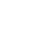Live chat1. Financial markets are systems which enable people to buy and sell financial securities which can be either in stocks, bonds and or financial instruments. Financial markets operate at market value basis given the available information on the market.

The significant function of the financial markets is to make possible for agents to borrow or lend money by transferring securities from one individual to the other; this enables individuals facing financial constraints to be able to acquire liquidity in short periods. Therefore, financial markets offer liquidity in short notice. Financial markets collect information and analyze the information to determine whether it reflects on market prices and its effect on the prices. Usually when new stocks are listed in the financial markets, the fair market value is determined by the financial markets. Financial markets allow individuals to transfer risk from shareholders to investors and its operations are efficient since transactions require less time and reduced transaction costs.An economy would not have a venue to determine the actual value of stocks leading to overvaluation or undervaluation of securities. This would lead to adverse economic conditions. It would be difficult for investors to determine where and how to invest since market information is not readily available. Liquidity would not be easily obtained.

1. Capital markets are where securities are traded which require a maturity period of more than one financial year, whereas money markets is the component of the financial market that deals with financial securities for a period of one year or less.
2. The corporations have a ready market for buying and selling their securities. Valuation is determined by market forces and therefore, no unfair trading. Security exchanges facilitate expedient transfer of securities and hence liquidity issues are solved expediently.
3. Private placement facilitates capital to be raised faster than in public offers. In public offers full disclosure of information is required which is not the case in private offer hence expediting the availability of capital. Private placement does not require adhering to stringent rules and regulations posed by public offers; therefore, it is cheaper and cost effective.
4. Savings can be transferred directly to the business account, savings can be transferred through an intermediary who acts on behalf of the business firm and funds can be transferred through a financial institution as an intermediary.
5. (a)Breakeven point : sales = total costs

Selling price/unit = 85, variable cost/ unit = 58, fixed cost /year =170000.

Limited time Offer

0
0
days
:
0
0
hours
:
0
0
minutes
:
0
0
seconds
Get 19% OFF

Let y be no of units at breakeven point. Therefore, 58y+170000=85y; 85y-58y=170000; 27y=170000; y=6296.3. Therefore, breakeven point in units is 6296.3 units

(b) Dollar sales volume = 6296.3*85=535,185.5

(c) profit/loss at 7000 units of production. Sales = 7000*85=595,000; variable costs= 7000*58=406,000; fixed cost=170000; profit/loss=sales-total costs. Therefore, 595000-(406,000+170,000) =19000. (Profit)

Profit/loss at 9000 units of production, sales =9000*85=765,000; variable costs= 9000*58=522,000; fixed cost=170000: therefore, 765,000-(522000+170000) =73000(profit)

Profit/loss at 15000 units of production, sales=85*15000=1,275,000; variable cost=58*15000=870,000; fixed costs 170,000: therefore, 1275000-(870,000+170,000) =235,000(profit)

(d) Degree of operating leverage =total costs of production/fixed costs of production.

At 7000 units of production, total costs =variable costs + fixed costs; 406,000+170,000=576,000; operating leverage=576,000/170,000=3.4times

At 9000 units of production; total cost; 522,000+170,000=692,000; operating leverage=692,000/170,000=4.1times

At 15,000 units of production, total costs; 870,000+170,000=1,040, 000; operating leverage= 1,040,000/170,000=6.1 times.

1. Return on operating assets= net income/operating assets. Let y be net income. 16%=y/3.25million, therefore, y=0.16*3.25million=0.52million (net income)

Operating assets turnover= net sales/net assets. Let y be net sales. 6=y/3.25millions; y=6*3.25million; y =19.5million (net sales)

Stay ConnectedDegree of operating leverage =total cost/fixed costs. Total cost= sales –net income. Therefore, 19.5million-0.52million=18.98million (total cost)

Let fixed costs be y. 18.98million/y=9; 9y=18.98million; y=18.98million/9=2.11million (fixed costs)

Selling price/ unit=19.5million/1.7million units=\$11.47(selling price per unit)

Variable cost/unit=18.98million – 2.11million=16.87million; 16.87million/1.7million=\$9.9 (variable cost per unit)

Breakeven point, sales=total costs; let y be the units at breakeven point. 11.47y=9.9y+2.11; 11.47y-9.9y=2.11; =1.57y=2.11; y=2.11/1.57=1.34; therefore, breakeven point in units =1.34million units.

0

Preparing Orders

0

Active Writers

0%

Positive Feedback

0

Support Agents

Now Accepting Apple Pay!
Limited offerget 15% off your 1st order with code first15